DownloadDocumentationHNS → Hypercomplex number systems (HNS)

## Hypercomplex number systems (HNS)

On the modern stage of mathematical simulation development, information presentation by the help of HNS has several advantages enabling to provide simulation efficiency.
Above all things, at HNS application, the peculiarities of object design structure are taken into account. For example, at designing tasks connected with motion in space, application of quaternions considerably improves the calculating procedure and the results received. In many cases of HNS application for simulation, dimension of sets of equations which describe the objects, is decreased. This gives the possibility of using analytical calculations at simulation, which also increases efficiency of the latter.

Hypercomplex numerical system of n dimension is set of numbers likewith operations of addition and multiplication introduced by certain laws.
Aggregate of elements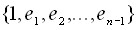is HNS basis. To define multiplication operations and full presentation of hypercomplex number system, it is necessary to define the rules of basis elements multiplication. Basic requirement to this operation is that, set of numbers must be closed against this operation:,
where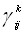coefficients are structural constants of the system. The multiplication of base elements is set in a table. For example, table for complex numbers looks in the following way:
< /tr>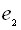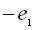To reduce the amount of calculations at mathematical simulation, it is reasonable to consider canonical HNS proposed by Shtudy, in which for every multiplicationeither allequal zero, or one of them is nonzero, and equals.
Two main operations are introduced in HNS, namely addition and multiplication:,and zero and unit elements.

Depending on composition law properties, set of HNS
disintegrates into the line of classes (fig. 1):Fig.1. HNS classification.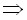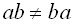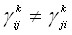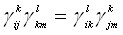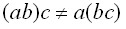The examples of commutative HNS are systems of complex, dual, double quadriplex and biquadriplex numbers; the eaxmples of noncommutative HNS are quaternions, octaves, Pauli numbers.

The infinite set of HNS, which differ in dimension and basic elements composition laws, can be built. Properties of different HNS can differ substantially. There are such criteria by which it is possible to classify HNS: dimension; belonging to the class of isomorphism; composition law properties; structural properties; canonicity; presence of unit element in the basis; presence of zero divisors.